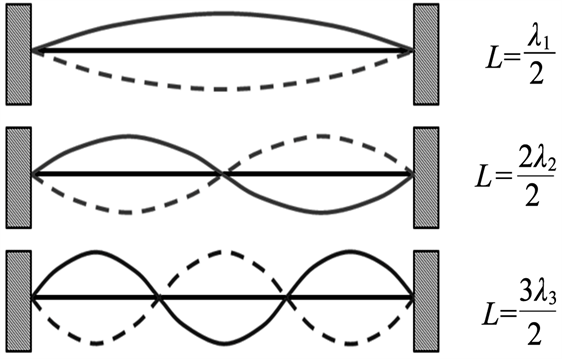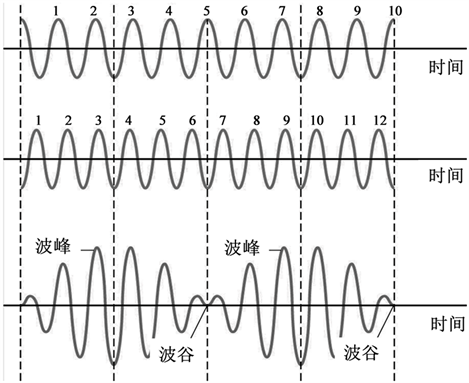# 乐器发声的基本物理原理The Basic Physical Principles of Vocal Instrument

• 全文下载: PDF(592KB)    PP.336-341   DOI: 10.12677/APP.2018.87043
• 下载量: 809  浏览量: 4,709

Musical instruments can be divided into stringed instruments, wind instruments and percussion instruments and other types. Because the sound principle of musical instrument is different, the physics principle behind it is also different. In this paper, the classification and corresponding principles of musical instruments are introduced, and some basic physical principles are systematically explained, and then the physical principles of vocal sounds of several typical musical instruments are analyzed in detail.

1. 引言

2. 乐器发声的基本物理原理

2.1. 拉紧弦的波速公式

$v=\sqrt{\frac{{F}_{T}}{\mu }}$ (1)

2.2. 弦的驻波解Figure 1. Solution of standing wave with fixed chord

$L=n\frac{\lambda }{2}$ (2)

${f}_{n}=n{f}_{1}$ (3)

2.3. 乐音(或拍频)

$\begin{array}{l}{x}_{1}=A\mathrm{cos}2\text{π}{f}_{1}t\\ {x}_{2}=A\mathrm{cos}2\text{π}{f}_{2}t\end{array}$ (4)

$x={x}_{1}+{x}_{2}=2A\mathrm{cos}\left[\text{π}\left({f}_{1}-{f}_{2}\right)t\right]\mathrm{cos}\left[\text{π}\left({f}_{1}+{f}_{2}\right)t\right]$ (5)

${f}_{b}=|{f}_{1}-{f}_{2}|$ (6)

2.4. 声波的谐振(共鸣)

1) 封闭管：封闭管中声波需要经过一个往返形成半个波长的波程差，形成共鸣。这样，形成驻波的最大波长是：

$\lambda =4L$ (7)

2) 开放管：开放管中声波需要经过半个波长的波程差，形成共鸣。这样，形成驻波的最大波长是：

$\lambda =2L$ (8)Figure 2. Schematic diagram of beat frequency formationFigure 3. Propagation of sound waves in closed tubes

2.5. 其他经验公式

1) 声波在空气中的传播速度与温度有关，温度越高，传播速度越大。以摄氏为例，0℃时，声速约为331 m/s，温度每升高1℃，速度增加0.6 m/s，可用公式(9)表示。

$v\left(t\right)=v\left(0\right)\left(1+\alpha t\right)$ (9)

2) 打击乐器中板的振动频率。由于板可以看为二维刚体，其振动频率受板的形状、刚性、密度、尺寸和敲打方式等多重因素决定，其基频公式就是一个很复杂的形式，见公式(10)  。

${f}_{mn}=\frac{\text{π}h}{2{r}^{2}}\sqrt{\frac{E}{3\rho \left(1-{\mu }^{2}\right)}}{\left({\beta }^{mn}\right)}^{2}$ (10)

3. 几种典型乐器发声的基本物理原理

3.1. 弦乐器

3.2. 管乐器

3.3. 打击乐器

4. 结束语

NOTES

*第一作者。

#通讯作者

  马文蔚. 大学物理[M]. 第6版. 北京: 高等教育出版社, 2012.  龚镇雄. 音乐与物理[J]. 物理, 1995, 24(9): 543-547.  马惠英, 佘守宪. 管中的驻波: 管乐器和簧乐器——物理与音乐之三[J]. 物理通报, 2004(4): 42-45.  马惠英, 佘守宪. 打击乐器和膜、棒、板的振动——物理与音乐专题之四[J]. 物理通报, 2004(5): 43-46.  Valiaki, V. (2004) Physics-Based Modeling of Musical Instruments. Acta Acustica United with Acustica, 90, 611-617.